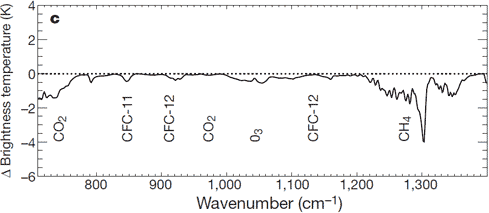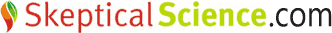# Quantifying the human contribution to global warming

The amount of warming caused by the anthropogenic increase in atmospheric CO2 may be one of the most misunderstood subjects in climate science. Many people think the anthropogenic warming can't be quantified, many others think it must be an insignificant amount. However, climate scientists have indeed quantified the anthropogenic contribution to global warming using empirical observations and fundamental physical equations.Figure 1: Carbon dioxide concentrations in the atmosphere over both the last 1000 years and the preceding 400,000 years as measured in ice cores

As a greenhouse gas, this increase in atmospheric CO2 increases the amount of downward longwave radiation from the atmosphere, including towards the Earth's surface.

### Surface measurements of downward longwave radiation

The increase in atmospheric CO2 and other greenhouse gases has increased the amount of infrared radiation absorbed and re-emitted by these molecules in the atmosphere. The Earth receives energy from the Sun in the form of visible light and ultraviolet radiation, which is then re-radiated away from the surface as thermal radiation in infrared wavelengths. Some of this thermal radiation is then absorbed by greenhouse gases in the atmosphere and re-emitted in all directions, some back downwards, increasing the amount of energy bombarding the Earth's surface. This increase in downward infrared radiation has been observed through spectroscopy, which measures changes in the electromagnetic spectrum.Figure 2: Spectrum of the greenhouse radiation measured at the surface. Greenhouse effect from water vapor is filtered out, showing the contributions of other greenhouse gases (Evans 2006).

### Satellite measurements of outgoing longwave radiation

The increased greenhouse effect is also confirmed by NASA's IRIS satellite and the Japanese Space Agency's IMG satellite observing less longwave leaving the Earth's atmosphere.Figure 3: Change in spectrum from 1970 to 1996 due to trace gases. 'Brightness temperature' indicates equivalent blackbody temperature (Harries 2001).

The increased energy reaching the Earth's surface from the increased greenhouse effect causes it to warm. So how do we quantify the amount of warming that it causes?

Radiative transfer models use fundamental physical equations and observations to translate this increased downward radiation into a radiative forcing, which effectively tells us how much increased energy is reaching the Earth's surface. Studies have shown that these radiative transfer models match up with the observed increase in energy reaching the Earth's surface with very good accuracy (Puckrin 2004). Scientists can then derive a formula for calculating the radiative forcing based on the change in the amount of each greenhouse gas in the atmosphere (Myhre 1998). Each greenhouse gas has a different radiative forcing formula, but the most important is that of CO2:

dF = 5.35 ln(C/Co)

Where 'dF' is the radiative forcing in Watts per square meter, 'C' is the concentration of atmospheric CO2, and 'Co' is the reference CO2 concentration. Normally the value of Co is chosen at the pre-industrial concentration of 280 ppmv.

Now that we know how to calculate the radiative forcing associated with an increase in CO2, how do we determine the associated temperature change?

### Climate sensitivity

As the name suggests, climate sensitivity is an estimate of how sensitive the climate is to an increase in a radiative forcing. The climate sensitivity value tells us how much the planet will warm or cool in response to a given radiative forcing change. As you might guess, the temperature change is proportional to the change in the amount of energy reaching the Earth's surface (the radiative forcing), and the climate sensitivity is the coefficient of proportionality:

dT = ?*dF

Where 'dT' is the change in the Earth's average surface temperature, '?' is the climate sensitivity, usually with units in Kelvin or degrees Celsius per Watts per square meter (°C/[W/m2]), and 'dF' is the radiative forcing.

So now to calculate the change in temperature, we just need to know the climate sensitivity. Studies have given a possible range of values of 2-4.5°C warming for a doubling of CO2 (IPCC 2007). Using these values it's a simple task to put the climate sensitivity into the units we need, using the formulas above:

? = dT/dF = dT/(5.35 * ln)= [2 to 4.5°C]/3.7 = 0.54 to 1.2°C/(W/m2)

Using this range of possible climate sensitivity values, we can plug ? into the formulas above and calculate the expected temperature change. The atmospheric CO2 concentration as of 2010 is about 390 ppmv. This gives us the value for 'C', and for 'Co' we'll use the pre-industrial value of 280 ppmv.

dT = ?*dF = ? * 5.35 * ln(390/280) = 1.8 * ?

Plugging in our possible climate sensitivity values, this gives us an expected surface temperature change of about 1–2.2°C of global warming, with a most likely value of 1.4°C. However, this tells us the equilibrium temperature. In reality it takes a long time to heat up the oceans due to their thermal inertia. For this reason there is currently a planetary energy imbalance, and the surface has only warmed about 0.8°C. In other words, even if we were to immediately stop adding CO2 to the atmosphere, the planet would warm another ~0.6°C until it reached this new equilibrium state (confirmed by Hansen 2005). This is referred to as the 'warming in the pipeline'.

Of course this is just the temperature change we expect to observe from the CO2 radiative forcing. Humans cause numerous other radiative forcings, both positive (e.g. other greenhouse gases) and negative (e.g. sulfate aerosols which block sunlight). Fortunately, the negative and positive forcings are roughly equal and cancel each other out, and the natural forcings over the past half century have also been approximately zero (Meehl 2004), so the radiative forcing from CO2 alone gives us a good estimate as to how much we expect to see the Earth's surface temperature change.Figure 4: Radiative forcing estimates from the IPCC report

We can also calculate the most conservative possible temperature change in response to the CO2 increase. Some climate scientists who are touted as 'skeptics' have suggested the actual climate sensitivity could be closer to 1°C for a doubling of CO2, or 0.27°C/(W/m2). Although numerous studies have ruled out climate sensitivity values this low, it's worth calculating how much of a temperature change this unrealistically low value would generate. Using the same formulas as above,

dT = 1.8 * ? = 1.8 * 0.27 = 0.5°C.

Therefore, even under this ultra-conservative unrealistic low climate sensitivity scenario, the increase in atmospheric CO2 over the past 150 years would account for over half of the observed 0.8°C increase in surface temperature.

NOTE: This post is the Advanced version (written by dana1981) of the skeptic argument "CO2 effect is weak" (note the nifty black diamond to denote the harder level). He adapted it from his Green Options article How Much Global Warming Are Humans Causing. If other climate bloggers are interested in allowing their existing articles to be used as advanced rebuttals to skeptic arguments, please contact me - I'd love to talk with you!

Posted by dana1981 on Friday, 3 September, 2010The Skeptical Science website by Skeptical Science is licensed under a Creative Commons Attribution 3.0 Unported License.